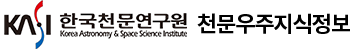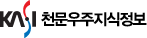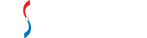#통합검색

#### 통합검색

인기검색어### 한국우주과학회지

• 홈으로 이동1984년 ~ 2019년까지 1,111 건한국우주과학회지를 계간으로 확인하실 수 있습니다.

• The Korean Space Science Society (The Korean Astronomical Society)
• 계간 (Quarterly)
• ISSN : 1225-052X (ISSN : 1225-052X)
• DB구축현황 : 1,111건 (DB Construction : 1,111 Articles)
안내사항
총 게시글 1,111 페이지 111/112
1101
• Kim, Sug-Whan
• 韓國宇宙科學會誌 = Journal of astronomy & space sciences
• 2, n.2
• pp.101-115
• 1985
• 원문 바로보기
Using Brandt model the mess distribution of the late type spiral galaxy NGC 6946 was derived, and the total mass was reestimated to understand the M/L ratio of this galaxy. Two kinds of the rotation curve with shape parameter n=1 and 3.3 were examined. The following are the main results; (1) The total masses of NGC 6946 are $3.1\times$ $10^{11}$ M(n=1) AND $2.8\times$ $10^{11}$ M(n=3.3) respectively. and the corresponding M/L are about 17 and 16 for both cases. (2) The optical image in the blue light, whose radius is 9.6 kpc, has 8 $\times$ $10^{10}$ M and 1.4 $\times$ $10^{11}$ M. These give the value of M/L about 5 and 8 respectively. (3) The masses and M/L of the nuclear region within 1.2 kpc are 4.0 $\times$ $10^{9}$ M 4.7 $\times$ $10^9$ M and 3, 4 for both cases. Those of the disk from 1.2 kpc to 9.6 kpc are 7.6 $\times$ 1 $10^{10}$ M, 1.4 $\times$ $10^{11}$ M, and 5, 8. (4) The masses of the outer halo extended to few hundreds kiloparsecs are 2.3 $\times$ $10^{11}$ M and 1.4 $\times$ $10^{11}$ M. The corresponding M/L are about 62 and 37.
1102
• Kim, Chul-Hee
• 韓國宇宙科學會誌 = Journal of astronomy & space sciences
• 2, n.2
• pp.131-151
• 1985
• 원문 바로보기
Fundamental data for 400 classical cepheids have been compiled. The PC-relation is derived and compared with similar relations for the Magellanic Clouds; it is fund that the differences are not great.
1103
• Kim, Ho-Il
• 韓國宇宙科學會誌 = Journal of astronomy & space sciences
• 2, n.1
• pp.35-45
• 1985
• 원문 바로보기
Standardized B and V photoelectric light curves of Algol are made with the obsevations obtained during 1982-84 with the 40-cm and the 61-cm reflectors of Yonsei University Observatory. These light curves show asymmetry between ascending and descending shoulders. The ascending shoulder is 0.02 mag brighter than descending shoulder in V light curve and 0.03mag in B light curve. These asymmetric light crves are interpreted as the result of inhomogeneous energy distribution on the surface of one star of the eclipsing pair rather than the result of gaseous stream flowing from KOIV to B8V star. The 180-year periodicity, so called great inequality, are most likely the result proposed by Kim et al.(1983) that the abrupt and discrete mass losses of cooler component may be the cause of this orbital change. The amount of mass loss deduced from these discrete period changes turned out to be of the order of $10^{-6}$ - $10^{-5}M_\odot$ .
1104
• 홍정호
• 韓國宇宙科學會誌 = Journal of astronomy & space sciences
• 2, n.2
• pp.117-129
• 1985
• 원문 바로보기
Holmberg의 은하 직정과 SUGC에 있는 de Vaucouleurs face-on 은하 직경과의 차이는 BGC와 Holmberg 목록으로 Heidmann 등이 구한 차이보다 더 적게 나타났는데, 이는 펑균표면광도 $\mu_B$ = 24.5 24.5 등급에서 구한 face-on 은하 직경을 수록한 BGC 자료들을 H eidmann 등이 사용했기 때문이다. 또, 본 논문에서 구한 Holmberg 은하 직경과 de Vaucouleurs은하 직경과의 상관관계식에서 $D_h>D_v$ 로 나타났으므로 Holmberg 은하 반청 $b=\frac{1}{2}D_v$ 로 사용하는것이 옳지 않다고 본다.
1105
• Nha, Il-Seong
• 韓國宇宙科學會誌 = Journal of astronomy & space sciences
• 2, n.2
• pp.69-99
• 1985
• 원문 바로보기
The UBV observations of the Brightest RS CVn-type eclipsing binary star AR Lac were made at four observatories, two in Korean and two in the U.S. in the 1980-81 and 1981-82 seasons. As a result of the cooperation, two light curves in the yellow and in the blue were completed for each observing seasons. Ultraviolet observations were also made at three of the four observatories. The orbital period of AR Lac apparantly decreased around 1977. An analysis of our yellow light curves together with five other yellow curves available in the literature since 1975 shows that there seems no periodicity in the migration of the distortion waves. There is a gradual decrease of at least 0.m1 between 1976 and 1982 in the brightness of the cooler component if one assumes that the hotter component is constant.
1106
• 김선규
• 韓國宇宙科學會誌 = Journal of astronomy & space sciences
• 1, n.1
• pp.35-39
• 1984
• 원문 바로보기
Space Shuttle의 성공적인 비행을 가능케한 요인중의 하나가 Silica Tile을 사용한 재사용 할 수 있는 Thermal Protection System의 설계이었다. 이러한 Thermal Protection System의 기본 설계 개념을 밝히고 또한 Silica Tile의 생산 공정과 Tile System의 구조를 서술하였다.
1107
• Choi, Kyu-Hong
• 韓國宇宙科學會誌 = Journal of astronomy & space sciences
• 1, n.1
• pp.23-34
• 1984
• 원문 바로보기
A bubble trapped in the liquid manifold of INTELSAT IV F-7 spacecraft caused a mass imbalance between the System 1 propellant tanks and a wobble half angle of 0.38 degree to 0.48 degree. A maneuver on May 14, 1980 passed the bubble through the axial jet and allowed propellant to redistribute. A 0.2 rpm change in sin rate was observed with an exponential decay time constant of 6 minutes. In this paper, moment of inertia, tank geometry and hydrodynamic models are derived to match the observed spin rate data. The values of the total mass of propellant considered were 16, 19 and 20 kgs with corresponding mass imbalances of 14.3, 15 and 15.1 Kgs, respectively. The result shows excellent agreement with observed spin rate data but it was necessary to assume a greater mass of hydrazine in the tanks than propellant accounting indicated.
1108
• Han, Won-Yong
• 韓國宇宙科學會誌 = Journal of astronomy & space sciences
• 1, n.1
• pp.41-53
• 1984
• 원문 바로보기
Photoelectric observations of the close eclipsing binary system CW Cep, which is well known of its apsidal motion, were made on 20 nights during August and November in 1983 using two 61cm reflectors at the Sobaeksan Observing Station of Korean National Astronomical Observatory and I1san Observing Station of Yonsei University Observatory. Standardized new light curves in UBV system are presented with a total of 1,422 individual observations. For the corrections of regional and instrumental differences, same standard stars were observed at the two observatories. Four new times of minimum light were determined with the method of Kwee and van Woerden(1956). With all of the collected times of minima, apsidal motion of this system was checked, but the O-C values calculated by the light elements of Nha(1975) and Soderhjelm (1976) did not coincide well with new times of minima. New light elements which satisfy most times of minima better, and are deduced the apsidal period derived by the new light elements turns out to be 43 years, somewhat longer than those of values previously known.
1109
• Lee, Yong-Sam
• 韓國宇宙科學會誌 = Journal of astronomy & space sciences
• 1, n.1
• pp.55-65
• 1984
• 원문 바로보기
A total of 618 photoelectric observations (302 in yellow and 316 in bule) is made in 1982 at the Yonsei University Observatory. Except that of the secondary eclipse the homogeneous coverage of observations successfully secured B, V, and B-V light curves. Enhanced distortions in the light curves are appeared at the phases aroung $0.^P1$ , which supports the wave-like migration period of 9.7 years(Rodono 1981). One epoch time of the primary minimum was made by combining the observations in three nights. This minimum time shows that the O-C values are still decreasing and there seems to indication of increasing. B and V light curves of the primary minimum are in strong asymmetry which show less luminous in the third and fourth contacts compared to those of the first and second ones. This asymmetry may be as a result of the reflection of the wave minimum at $0.^P1$ , and B-V curve also shows asymmetry, redder at the third contact than the second one by about $0.^m04$ . This color difference apperature distribution on the surface of cooler, larger component(KO IV star).
1110
• Chou, Kyong-Chol
• 韓國宇宙科學會誌 = Journal of astronomy & space sciences
• 1, n.1
• pp.5-21
• 1984
• 원문 바로보기
The airflow along the surface of a launch vehicle together with base flow of clustered nozzles cause problems which may affect the stability or efficiency of the entire vehicle. The problem may occur when the vehicle is on the launching pad or even during flight. As for such problems, local steady-state loads, overall steady-state loads, buffet, ground wind loads, base heating and rocket-nozzle hinge moments are examined here specifically.